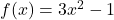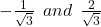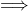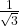## verify whether the values of x given in each case are the zeroes of the polynomial or not ?If the

Question

verify whether the values of x given in each case are the zeroes of the polynomial or not ?If the value of x =in progress 0
6 months 2021-08-15T15:54:58+00:00 1 Answers 0 views 0

## Answers ( )

INFORMATION :-the zeroes of a polynomial also called its roots are the values of x for which the corresponding value of y {f(x)} is zero.while seeing zeroes in a graph they are the points where the graph intersects the x- axis.

SOLUTION :-

Now, we’re given a function such that

f(x) = 3x²- 1

put f(x) = 0 and solve for x to get the zeroes of this function.

f(x) = 3x² – 1 = 0

3x² – 1 = 0

3x² = 1

x² =x = ±so the roots of f(x) = 3x²- 1 are :-

• +•Comparing the values given to us and the values we found out.

• 1st value is a zero of the function

while the

• 2nd value isn’t.